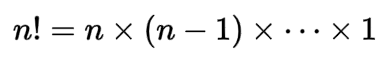Request a Tool

Factorial Calculator

Factorial Calculator is use to calculate factorial of input number.

Factorial
0

Formula• n! = Factorial of n value
• n = Value of n

Defination / Uses

The product of all positive integers less than or equal to n, indicated by n! in mathematics, is the factorial of a non-negative integer n: Consider the following illustration: According to standard practise, the value of 0! is 1.

A factorial is a function that multiplies a number by all subsequent numbers. 5!= 5*4*3*2*1=120, for example. The function is used to determine the number of ways “n” objects can be organised, among other things. The factorial is the product of all positive integers that are less than or equal to zero, according to the factorial definition. 0! has a factororial value of 1. The Factorial Sequence Calculator works on the same idea as the Factorial Sequence Calculator. The factorial notation calculator makes it simple to digitally calculate factorial variables. The factororial expression calculator gives instantaneous and reliable answers. You may also locate a thorough factorial tutorial if you want to understand more about how it works.